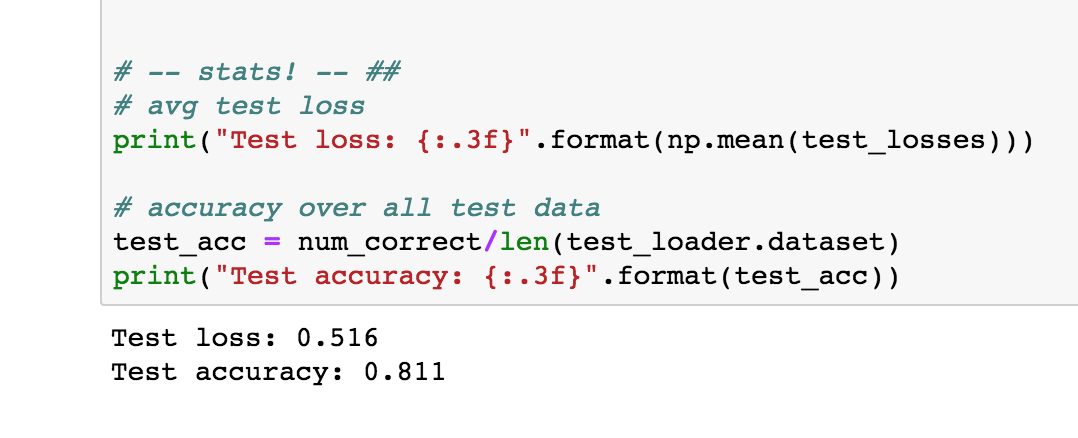# AI For Trading: Testing the Trained Model (103)

## Testing the Trained Model

I want to show you two great ways to test: using test data and using inference. The first is similar to what you’ve seen in our CNN lessons. I am iterating through the test data in the test_loader, recording the test loss and calculating the accuracy based on how many labels this model got correct!

I’m doing this by looking at the rounded value of our output. Recall that this is a sigmoid output between 0-1 and so rounding this value will give us an integer that is the most likely label: 0 or 1. Then I’m comparing that predicted label to the true label; if it matches, I record that as a correctly-labeled test review.

# Get test data loss and accuracy

test_losses = [] # track loss
num_correct = 0

# init hidden state
h = net.init_hidden(batch_size)

net.eval()
# iterate over test data

# Creating new variables for the hidden state, otherwise
# we'd backprop through the entire training history
h = tuple([each.data for each in h])

if(train_on_gpu):
inputs, labels = inputs.cuda(), labels.cuda()

# get predicted outputs
output, h = net(inputs, h)

# calculate loss
test_loss = criterion(output.squeeze(), labels.float())
test_losses.append(test_loss.item())

# convert output probabilities to predicted class (0 or 1)
pred = torch.round(output.squeeze())  # rounds to the nearest integer

# compare predictions to true label
correct_tensor = pred.eq(labels.float().view_as(pred))
correct = np.squeeze(correct_tensor.numpy()) if not train_on_gpu else np.squeeze(correct_tensor.cpu().numpy())
num_correct += np.sum(correct)

# -- stats! -- ##
# avg test loss
print("Test loss: {:.3f}".format(np.mean(test_losses)))

# accuracy over all test data
print("Test accuracy: {:.3f}".format(test_acc))

Below, I’m printing out the average test loss and the accuracy, which is just the number of correctly classified items divided by the number of pieces of test data,total.

We can see that the test loss is 0.516and the accuracy is about 81.1% !Test results

Next, you're ready for your last task! Which is to define a predict function to perform inference on any given text review!

### Exercise: Inference on a test review

You can change this test_review to any text that you want. Read it and think: is it pos or neg? Then see if your model predicts correctly!

Exercise: Write a predict function that takes in a trained net, a plain text_review, and a sequence length, and prints out a custom statement for a positive or negative review!

You can use any functions that you've already defined or define any helper functions you want to complete predict, but it should just take in a trained net, a text review, and a sequence length.

def predict(net, test_review, sequence_length=200):
''' Prints out whether a give review is predicted to be
positive or negative in sentiment, using a trained model.

params:
net - A trained net
test_review - a review made of normal text and punctuation
sequence_length - the padded length of a review
'''

# print custom response based on whether test_review is pos/neg

Try to solve this task on your own, then check out the solution, next!